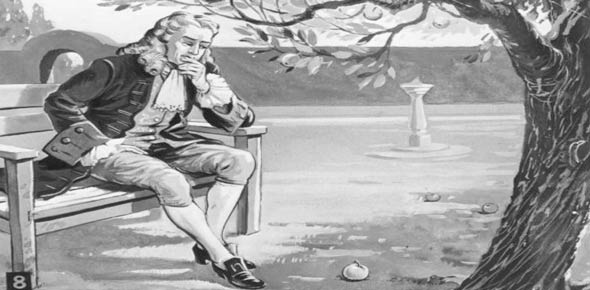# Trivia Quiz On Newton's Laws Of Motion

5 Questions | Total Attempts: 102SettingsIn this test you we be tested on The laws of Motion.

Related Topics
• 1.
What is the first Law of Motion?
• A.

The Law of Acceleration

• B.

The Law of Inertia

• C.

The Law Reciprocal Action

• 2.
"For every action, there is an equal and opposite reaction" is what law of motion
• A.

The Law of Acceleration

• B.

The Law of Inertia

• C.

The Law Reciprocal Action

• 3.
Mass x Acceleration=
• A.

Gravity

• B.

Weight

• C.

Force

• 4.
Who discoverd the Laws of Motion?
• A.

Mrs. Fox

• B.

Mr. Fair

• C.

Sir Isaac Newton

• 5.
What law was demonstrated during the use of your balloon car?
• A.

The Law of Acceleration

• B.

The Law Reciprocal Action

• C.

The Law of Inertia